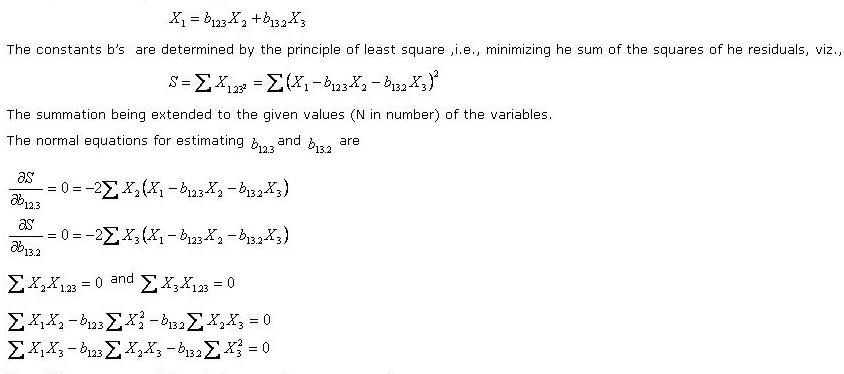# Statistics Assignment Help With Plane Of regression

## 8.12 Plane Of regression:The equation of the plane of regression of X1 on X2 and X3 is

###Since Xi's are measured from their respective means, we have